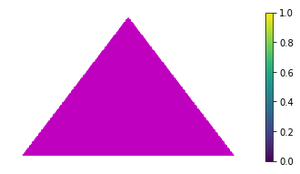# How to change Matplotlib color bar size in Python?

• Last Updated : 03 Jan, 2021

Colorbar is a separate axis that provides a current colormap indicating mapping of data-points into colors. In this article, We are going to change Matplotlib color bar size in Python. There are several ways with which you can resize your color-bar or adjust its position. Let’s see it one by one.

Method 1: Resizing color-bar using shrink keyword argument

Using the shrink attribute of colorbar() function we can scale the size of the colorbar.

Syntax : matplotlib.pyplot.colorbar(mappable=None, shrink=scale)

Basically, we are multiplying by some factor to the original size of the color-bar. In the below example by using 0.5 as a factor, We are having the original color-bar size.

Example 1:

## Python3

 `# importing library ` `import` `matplotlib.pyplot as plt ` ` `  `# Some data to show as an image ` `data ``=` `[[``1``, ``2``, ``3``], ` `        ``[``4``, ``5``, ``6``]] ` ` `  `# Call imshow() to display 2-D data as an image ` `img ``=` `plt.imshow(data) ` ` `  `# Scaling colorbar by factor 0.5 ` `plt.colorbar(shrink``=``0.5``) ` `plt.show() `

Output:Using_shrink_attribute

Example 2: In this example, we are using factor 0.75. Similarly, you can use any factor to change the color-bar size. The default value of the shrink attribute is 1.

## Python3

 `import` `matplotlib.pyplot as plt ` `import` `matplotlib.image as mpimg ` `fig, ax ``=` `plt.subplots() ` ` `  `# getting rid of axis ` `plt.axis(``'off'``) ` ` `  `# Reading saved image from folder ` `img ``=` `mpimg.imread(r``'img.jpg'``) ` ` `  `# displaying image ` `plt.imshow(img) ` ` `  `# Scaling by factor 0.75 ` `plt.colorbar(shrink``=``0.75``) ` `plt.show() `

Output:Using_shrink_attribute

Method 2: Using AxesDivider class

With this class, you can change the size of the colorbar axes however height will be the same as the current axes. Here we are using axes_divider.make_axes_locatable function which returns the AxisDivider object for our current axes in which our image is shown.

Example:

## Python3

 `# importing libraries ` `import` `matplotlib.pyplot as plt ` `from` `mpl_toolkits.axes_grid1 ``import` `make_axes_locatable ` ` `  `fig, ax ``=` `plt.subplots() ` `# Reading image from folder ` ` `  `img ``=` `mpimg.imread(r``'img.jpg'``) ` `image ``=` `plt.imshow(img) ` ` `  `# Locating current axes ` `divider ``=` `make_axes_locatable(ax) ` ` `  `# creating new axes on the right ` `# side of current axes(ax). ` `# The width of cax will be 5% of ax ` `# and the padding between cax and ax ` `# will be fixed at 0.05 inch. ` `colorbar_axes ``=` `divider.append_axes(``"right"``, ` `                                    ``size``=``"10%"``, ` `                                    ``pad``=``0.1``) ` `# Using new axes for colorbar ` `plt.colorbar(image, cax``=``colorbar_axes) ` `plt.show() `

Output:Using_AxisDivider

My Personal Notes arrow_drop_up
Recommended Articles
Page :